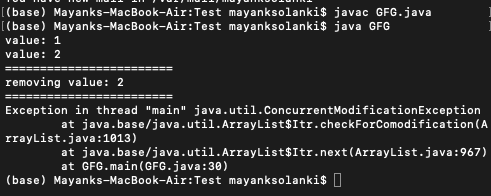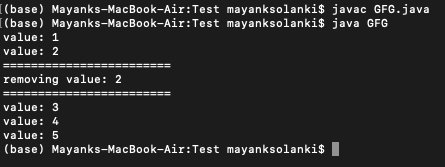GFG App
Open AppBrowser
Continue

# ConcurrentModificationException while using Iterator in Java

ConcurrentModificationException has thrown by methods that have detected concurrent modification of an object when such modification is not permissible. If a thread modifies a collection directly while it is iterating over the collection with a fail-fast iterator, the iterator will throw this ConcurrentModificationException. Here we will be understanding this exception with an example of why it occurs and how changes are made simultaneously which is the root cause for this exception. In the later part, we will understand how to fix it up.

Example 1: ConcurrentModificationException

## Java

 `// Java Program to ConcurrentModificationException while ` `// using Iterator ` ` `  `// Importing ArrayList and Iterator classes from java.util ` `// package ` `import` `java.util.ArrayList; ` `import` `java.util.Iterator; ` ` `  `// Main class ` `public` `class` `GFG { ` ` `  `    ``// Main driver method ` `    ``public` `static` `void` `main(String[] args) ` `    ``{ ` `        ``// Creating an object of ArrayList class ` `        ``// Declaring object of Integer type ` `        ``ArrayList list = ``new` `ArrayList<>(); ` ` `  `        ``// Adding custom integer elements to the object ` `        ``list.add(``1``); ` `        ``list.add(``2``); ` `        ``list.add(``3``); ` `        ``list.add(``4``); ` `        ``list.add(``5``); ` ` `  `        ``// Iterating over object elements using iterator ` `        ``Iterator iterator = list.iterator(); ` ` `  `        ``// Condition check ` `        ``// It holds true till there is single element ` `        ``// remainin in the List ` `        ``while` `(iterator.hasNext()) { ` ` `  `            ``// Rolling over to next element using next() ` `            ``// method ` `            ``Integer value = iterator.next(); ` ` `  `            ``// Print the element value ` `            ``System.out.println(``"value: "` `+ value); ` ` `  `            ``// If element equals certain value ` `            ``if` `(value.equals(``2``)) { ` ` `  `                ``// Display command for better readability ` `                ``System.out.println( ` `                    ``"========================"``); ` ` `  `                ``// Removing entered value in object ` `                ``System.out.println(``"removing value: "` `                                   ``+ value); ` ` `  `                ``// Making changes simultaneously ` `                ``System.out.println( ` `                    ``"========================"``); ` `                ``list.remove(value); ` `            ``} ` `        ``} ` `    ``} ` `}`

Output:Output Explanation:

ConcurrentModificationException is thrown when the next() method is called as the iterator is iterating the List, and we are making modifications in it simultaneously. Now in order to avoid this exception so let us do discuss a way out of using iterator directly which is as follows:

Example 2: Resolving ConcurrentModificationException

## Java

 `// Java Program to Avoid ConcurrentModificationException by ` `// directly using Iterator ` ` `  `// Importing ArrayList and Iterator classes ` `// from java.util package ` `import` `java.util.ArrayList; ` `import` `java.util.Iterator; ` ` `  `// Main class ` `public` `class` `Main { ` ` `  `    ``// Mai driver method ` `    ``public` `static` `void` `main(String[] args) ` `    ``{ ` `        ``// Creating an ArrayList object of integer type ` `        ``ArrayList list = ``new` `ArrayList<>(); ` ` `  `        ``// Custom integer elements are added ` `        ``list.add(``1``); ` `        ``list.add(``2``); ` `        ``list.add(``3``); ` `        ``list.add(``4``); ` `        ``list.add(``5``); ` ` `  `        ``// Iterating directly over elements of object ` `        ``Iterator iterator = list.iterator(); ` ` `  `        ``// Condition check ` `        ``// It holds true till there is single element ` `        ``// remaining in the List using hasNext() method ` `        ``while` `(iterator.hasNext()) { ` ` `  `            ``// Rolling over elements using next() method ` `            ``Integer value = iterator.next(); ` ` `  `            ``// print the values ` `            ``System.out.println(``"value: "` `+ value); ` ` `  `            ``// If value equals certain integer element ` `            ``// entered Say it be 2 ` `            ``if` `(value.equals(``2``)) { ` ` `  `                ``// Display command only ` `                ``System.out.println( ` `                    ``"========================"``); ` ` `  `                ``// Removing the entered value  ` `                ``System.out.println(``"removing value: "` `                                   ``+ value); ` `                `  `                ``// Display command only ` `                ``System.out.println( ` `                    ``"========================"``); ` `               `  `                ``// Removing current value in Collection ` `                ``// using remove() method  ` `                ``iterator.remove(); ` `            ``} ` `        ``} ` `    ``} ` `}`

Output:Output Explanation:

ConcurrentModificationException is not thrown because the remove() method does not cause a ConcurrentModificationException.

My Personal Notes arrow_drop_up